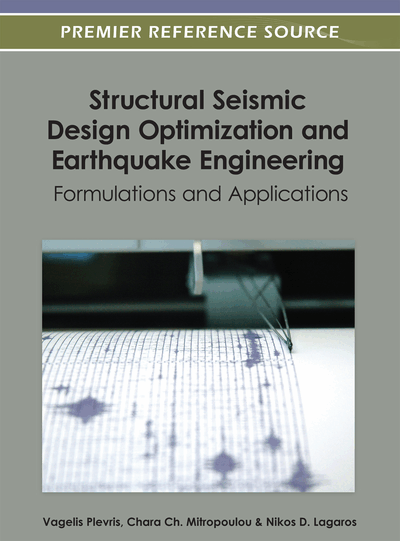# Performance-Based Seismic Design: A Search-Based Cost Optimization with Minimum Reliability Constraints

Oscar Möller (University of Rosario, Argentina), Marcelo Rubinstein (University of Rosario, Argentina), Fabián Savino (University of Rosario, Argentina) and Ricardo O. Foschi (University of British Columbia, Canada)
DOI: 10.4018/978-1-4666-1640-0.ch002

## Abstract

An approach is presented to structural optimization for performance-based design in earthquake engineering. The objective is the minimization of the total cost, including repairing damage produced by future earthquakes, and satisfying minimum target reliabilities in three performance levels (operational, life safety, and collapse). The different aspects of the method are considered: a nonlinear dynamic structural analysis to obtain responses for a set of earthquake records, representing these responses with neural networks, formulating limit-state functions in terms of deformations and damage, calculating achieved reliabilities to verify constraint violations, and the development of a gradient-free optimization algorithm. Two examples illustrate the methodology: 1) a reinforced concrete portal for which the design parameters are member dimensions and steel reinforcement ratios, and 2) optimization of the mass at the cap of a pile, to meet target reliabilities for two levels of cap displacement. The objective of this latter example is to illustrate model effects on optimization, using two different hysteresis approaches.
Chapter Preview
Top

## Introduction

Structural optimization for performance-based design in earthquake engineering aims at finding optimum design parameters (e.g.,dimensions of structural members, steel reinforcement ratios for concrete structures, characteristics of energy dissipation devices) corresponding to a minimum objective (e.g.,the total cost), with constraints specifying minimum reliability levels for each performance requirement. Three performance levels are normally considered in earthquake engineering: serviceability, life safety and collapse.

The objective of the present Chapter is to present a procedure for performance-based optimization of a preliminary design, taking into account the uncertainties in the ground motion and structural properties, and at the same time minimizing the total cost. This includes, in addition to the initial cost of construction, that associated with repairs following each of a series of earthquakes within a given time interval. The preliminary design may be the result of applying capacity design approaches, according to existing Codes, in order to satisfy the performance levels.

The subsequent sections of this Chapter discuss the different aspects of the proposed design optimization process, including: 1) the quantification of the seismic hazard or possible ground motions at the site; 2) the use of a structural dynamics analysis model that represents, as best as possible, the nonlinear response and the hysteretic energy dissipation mechanisms; 3) the calculation of a response database for the estimated ranges of the different intervening input random variables and design parameters; 4) a functional representation for the discrete databases, using neural networks response surfaces; 5) implementation of the response surfaces in the calculation of the reliability levels achieved, at each performance level, for specific values of the design parameters; 6) representation of these reliability levels by neural networks in terms of the design parameters, in order to achieve an efficient calculation of reliability constraint violations and, finally, 7) an optimization algorithm that would search for a minimum total cost objective under minimum reliability constraints for each performance level.

Earthquakes show large ground motion uncertainties which must be coupled with those in the structural capacities, and the demands will likely trigger a nonlinear structural response. For each performance level, the formulation of the limit-state functions requires the estimation of maximum responses (e.g., maximum inter-story drift) during the duration of the earthquake. Since only discrete values can be obtained by numerical analyses for specific combinations of the variables and parameters, the responses need to be given a continuous functional representation for optimization and reliability estimation. Each of the responses of interest can be approximately represented by using response surfaces which, when properly adjusted, can be used as substitutes for the dynamic analysis (Hurtado, 2004). Different forms for approximating surfaces have been studied (Möller et al., 2009b), and neural networks have been shown to offer advantages of flexibility and adaptability. Regardless of the type of response surface used, a major advantage of the substitute is the computational efficiency achieved with Monte Carlo-type simulations when estimating probabilities of non-performance.

The approach chosen in the optimization problem must consider the presence of constraints, the dimensionality and the form and number of objective functions. Optimization methods can use different approaches (Pérez López, 2005; Swisher et al., 2000), some requiring the calculation of gradients (steepest descent, conjugate gradients, Newton or quasi-Newton schemes) and others, not using gradients, implementing genetic or search algorithms. This Chapter proposes an efficient search-based algorithm which also accounts for constraints given by specified minimum reliability levels at each performance level. The optimization approach presented in this Chapter is based on previous work by the authors (Möller et al., 2007, 2008, 2009a,c), and introduces additional contributions as follows:

• The optimization process is started from a preliminary, deterministic seismic design;

• The previous search-based algorithm by the authors has been modified to minimize the possibility of encountering local minima;

• The damage repair costs introduced into the objective function take into account the occurrence of multiple seismic events during the life of the structure.

## Complete Chapter List

Search this Book:
Reset